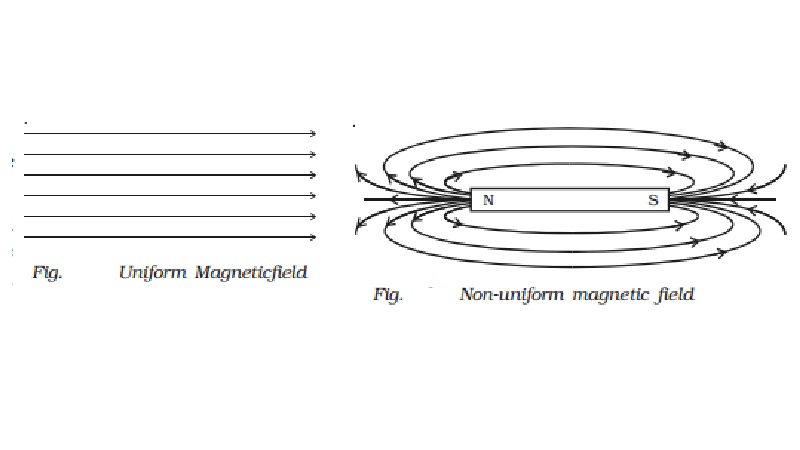Home | | Physics | | Physics | Basic properties of magnets

# Basic properties of magnetsWhen the magnet is dipped in iron filings, they cling to the ends of the magnet. The attraction is maximum at the two ends of the magnet. These ends are called poles of the magnet.

Basic properties of magnets

(i)When the magnet is dipped in iron filings, they cling to the ends of the magnet. The attraction is maximum at the two ends of the magnet. These ends are called poles of the magnet.

(ii) When a magnet is freely suspended, it always points along north-south direction. The pole pointing towards geographic north is called north pole N and the pole which points towards geographic south is called south pole S.

(iii) Magnetic poles always exist in pairs. (i.e) isolated magnetic pole does not exist.

The magnetic length of a magnet is always less than its geometric length, because the poles are situated a little inwards from the free ends of the magnet. (But for the purpose of calculation the  geometric length is always taken as magnetic length.)

(v)                   Like poles repel each other and unlike poles attract each other. North pole of a magnet when brought near north pole of another magnet, we can observe repulsion, but when the north pole of one magnet is brought near south pole of another magnet, we observe attraction.

(vi)The force of attraction or repulsion between two magnetic poles is given by Coulomb?s inverse square law.

Note : In recent days, the concept of magnetic poles has been completely changed. The origin of magnetism is traced only due to the flow of current. But anyhow, we have retained the conventional idea of magnetic poles in this chapter. Pole strength is denoted by m and its unit is ampere metre.

Magnetic moment

Since any magnet has two poles, it is also called a magnetic dipole.

The magnetic moment of a magnet is defined as the product of the pole strength and the distance between the two poles.

If m is the pole strength of each pole and 2l is the distance between the poles, the magnetic moment

Vector  M = m (2 Vector  l )

Magnetic moment is a vector quantity. It is denoted by M. Its unit is A m2. Its direction is from south pole to north pole.

Magnetic field

Magnetic field is the space in which a magnetic pole experiences a force or it is the space around a magnet in which the influence of the magnet is felt.

Magnetic induction

Magnetic induction is the fundamental character of a magnetic field at a point.

Magnetic induction at a point in a magnetic field is the force experienced by unit north pole placed at that point. It is denoted by B. Its unit is N/Am . It is a vector quantity. It is also called as magnetic flux density.

If a magnetic pole of strength m placed at a point in a magnetic field experiences a force F, the magnetic induction at that point is

B=F/m

Magnetic lines of force

A magnetic field is better studied by drawing as many number of magnetic lines of force as possible.

A magnetic line of force is a line along which a free isolated north pole would travel when it is placed in the magnetic field.

Properties of magnetic lines of force

(i)Magnetic lines of forces are closed continuous curves, extending through the body of the magnet.

(ii) The direction of line of force is from north pole to south pole outside the magnet while it is from south pole to north pole inside the magnet.

(iii)                The tangent to the magnetic line of force at any point gives the direction of magnetic field at that point. (i.e) it gives the direction of magnetic induction (Vector B ) at that point

(iv)                They never intersect each other.

(v) They crowd where the magnetic field is strong and thin out where the field is weak.

Magnetic flux and magnetic flux density

The number of magnetic lines of force passing through an area A is called magnetic flux. It is denoted by φ . Its unit is weber. It is a scalar quantity.

The number of magnetic lines of force crossing unit area kept normal to the direction of line of force is magnetic flux density. Its unit is Wb m?2 or tesla or N A?1m?1.

Magnetic flux  φ   =  Vector B . Vector  A

Uniform and non-uniform magnetic field

Magnetic field is said to be uniform if the magnetic induction has the same magnitude and the same direction at all the points in the region. It is represented by drawing parallel lines (Fig.).An example of uniform magnetic field over a wide area is the Earth?s magnetic field.

If the magnetic induction varies in magnitude and direction at different points in a region, the magnetic field is said to be non-uniform. The magnetic field due to a bar magnet is non-uniform. It is represented by convergent or divergent lines (Fig.).

Study Material, Lecturing Notes, Assignment, Reference, Wiki description explanation, brief detail

Related Topics